# The Opposite angles of Two Equal Sides of a Triangle are Equal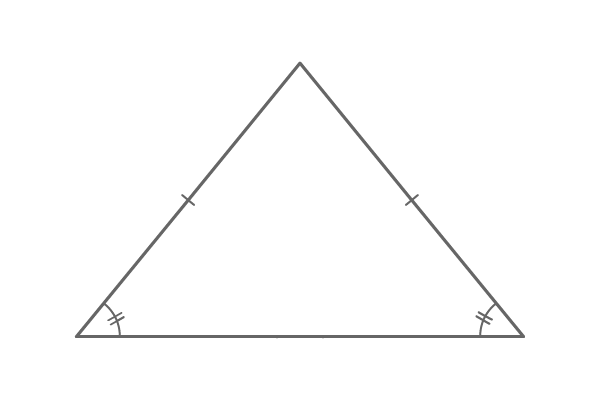## Theorem

The angles opposite to the two equal length sides in a triangle are equal.

The lengths of two sides of a triangle can be equal in some cases and it is possible in the case of isosceles triangle. The equality property of two sides make isosceles triangle to have symmetry property.

Hence, the angles opposite to the two equal length sides are always equal geometrically.

### Proof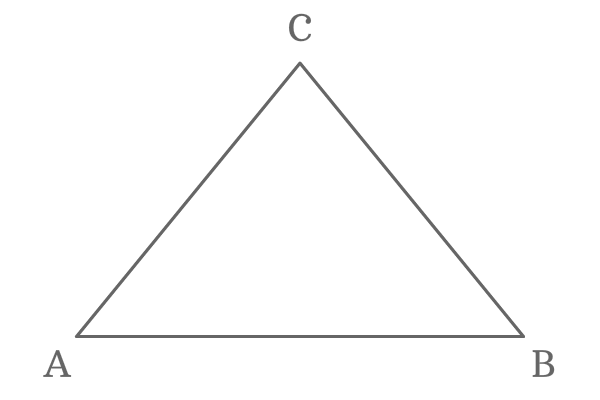$\Delta ABC$ is a triangle and its two sides are of equal length. Hence, the triangle $ABC$ is called an isosceles triangle. In this isosceles triangle, the lengths of the sides $\overline{AC}$ and $\overline{BC}$ are equal.

$AC = BC$

Draw a perpendicular line to the side $\overline{AB}$ from point $C$. It divides the side $\overline{AB}$ at its middle point exactly and the point of intersection is $D$.

Therefore, $AD = BD$.

Similarly, the line $\overline{DC}$ divides the $\Delta ABC$ as two right angled triangles $\Delta DAC$ and $\Delta DBC$. Now, the perpendicular line $\overline{DC}$ is a common side to both right angled triangles.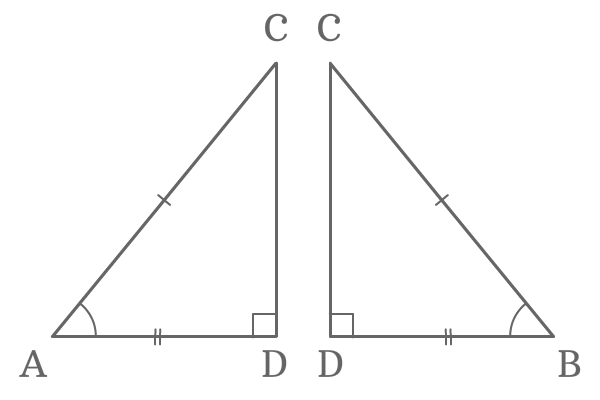Now, compare the lengths of three sides of both triangles.

1. $AC = BC$
2. $AD = BD$
3. $DC = DC$

The comparison of lengths of sides of the both triangles revealed that the two triangles are same but represented differently.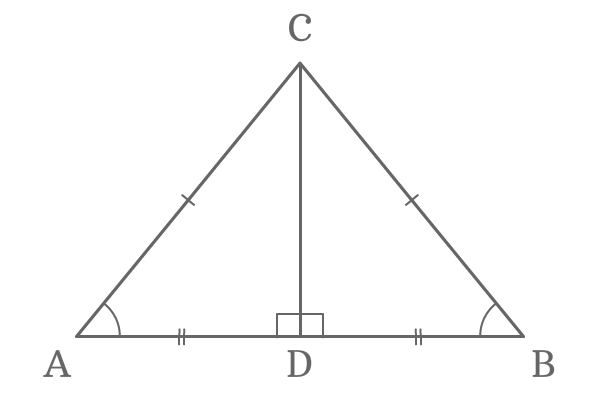Therefore, the $\Delta DAC$ and $\Delta DBC$ are known as congruent triangles and they also represent Right angle Hypotenuse side (RHS) criterion.

$\Delta DAC \cong \Delta DBC$

In this case, $\angle DAC$ is the opposite angle to the side $\overline{BC}$ and $\angle DBC$ is also opposite angle to the side $\overline{AC}$. The two angles are equals due to the congruency.

$\angle DAC = \angle DBC$

Therefore, if two sides of a triangle are equal, then the angles opposite to them are also equal.

#### Example

This property of the triangle can be proved geometrically by constructing a triangle but the lengths of any two sides of the triangle should be equal.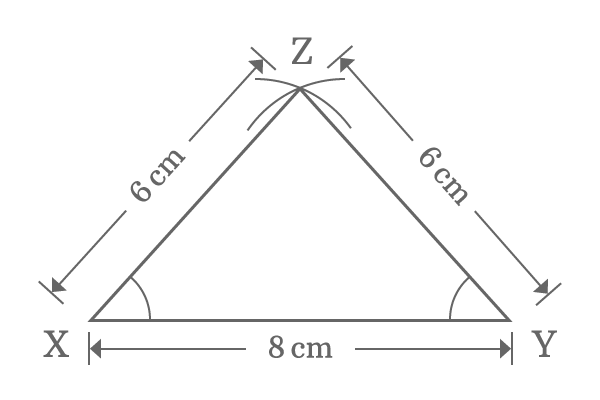Take ruler and draw a straight line ($\overline{XY}$) of length $8 \, cm$ horizontally.

Use compass and set distance between needle point and pencil point to $6 \, cm$. Draw an arc from point $X$ and also draw another arc from point $Y$ but the arcs should be intersected. The intersecting point of both arcs is $Z$.

Join points $X$ and $Z$ and also join points $Y$ and $Z$ by a straight line using ruler. Thus, the $\Delta XYZ$ is constructed geometrically.

$\angle XYZ$ and $\angle YXZ$ are opposite angles of the equal length sides $\overline{XZ}$ and $\overline{YZ}$ respectively. Now, measure the angles $XYZ$ and $YXZ$ by using protractor.

You practically observe that the $\angle XYZ$ and $\angle YXZ$ are equal and it is $48^\circ$ geometrically. Therefore, the angles opposite to the two equal length sides are equal geometrically.

Email subscription
Math Doubts is a best place to learn mathematics and from basics to advanced scientific level for students, teachers and researchers. Know more
Follow us on Social Media
###### Math Problems

Learn how to solve easy to difficult mathematics problems of all topics in various methods with step by step process and also maths questions for practising.

Learn more